Home Practice
For learners and parents For teachers and schools
Textbooks
Full catalogue
Pricing SupportLog in

We think you are located in United States. Is this correct?

# Test yourself now

High marks in science are the key to your success and future plans. Test yourself and learn more on Siyavula Practice.

## 8.7 Summary (EMCJJ)

• A ratio describes the relationship between two quantities which have the same units.

$x:y \quad \text{ or } \quad \frac{x}{y} \quad \text{ or } \quad x \enspace \text{ to } \enspace y$
• If two or more ratios are equal to each other $$\left( \frac{m}{n} = \frac{p}{q} \right)$$, then $$m$$ and $$n$$ are in the same proportion as $$p$$ and $$q$$.

• A polygon is a plane, closed shape consisting of three or more line segments.

• Triangles with equal heights have areas which are proportional to their bases.

• Triangles with equal bases and between the same parallel lines are equal in area.

• Triangles on the same side of the same base and equal in area lie between parallel lines.

• A line drawn parallel to one side of a triangle divides the other two sides of the triangle in the same proportion.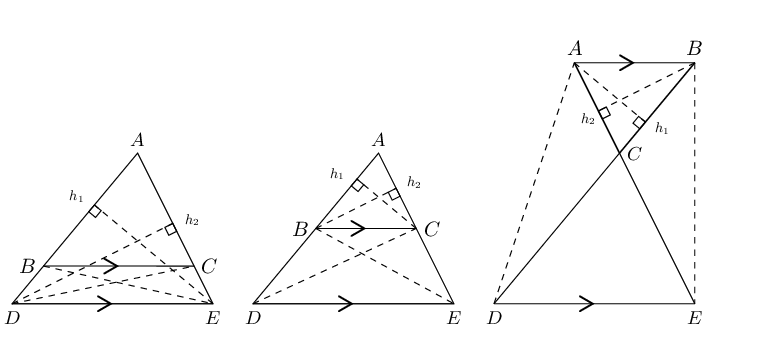• Converse: proportion theorem

If a line divides two sides of a triangle in the same proportion, then the line is parallel to the third side.

• Special case: the mid-point theorem

The line joining the mid-points of two sides of a triangle is parallel to the third side and equal to half the length of the third side.

If $$AB = BD$$ and $$AC = CE$$, then $$BC \parallel DE$$ and $$BC = \frac{1}{2}DE$$.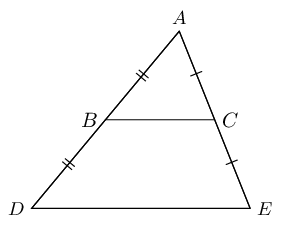• Converse: the mid-point theorem

The line drawn from the mid-point of one side of a triangle parallel to another side, bisects the third side of the triangle.

If $$AB = BD$$ and $$BC \parallel DE$$, then $$AC = CE$$.

• Polygons are similar if they are the same shape but differ in size. One polygon is an enlargement of the other.

• Two polygons with the same number of sides are similar when:

1. All pairs of corresponding angles are equal, and
2. All pairs of corresponding sides are in the same proportion.
• If two triangles are equiangular, then the triangles similar.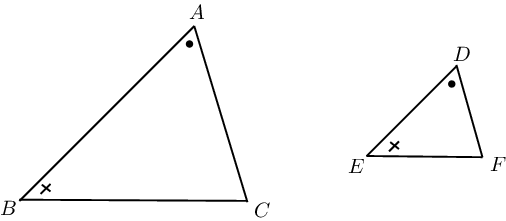• Triangles with sides in proportion are equiangular and therefore similar.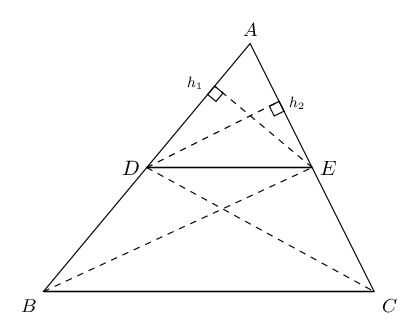• The square on the hypotenuse of a right-angled triangle is equal to the sum of the squares on the other two sides.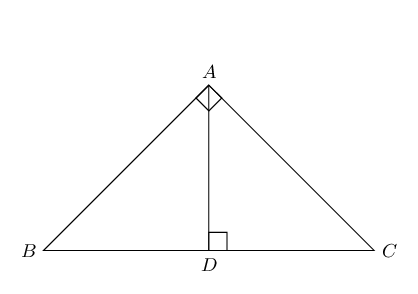• Converse: theorem of Pythagoras

If the square of one side of a triangle is equal to the sum of the squares of the other two sides of the triangle, then the angle included by these two sides is a right angle.